# MATLAB ONLY MATLAB ONLY MATLAB ONLY MATLAB ONLY MATLAB ONLY MATLAB ONLY MATLAB ONLY MATLAB ONLY....... Project V: Fourier Based System Modeling The last project in the class involves the modeling of a...

MATLAB ONLY MATLAB ONLY MATLAB ONLY MATLAB ONLY MATLAB ONLY MATLAB ONLY MATLAB ONLY MATLAB ONLY.......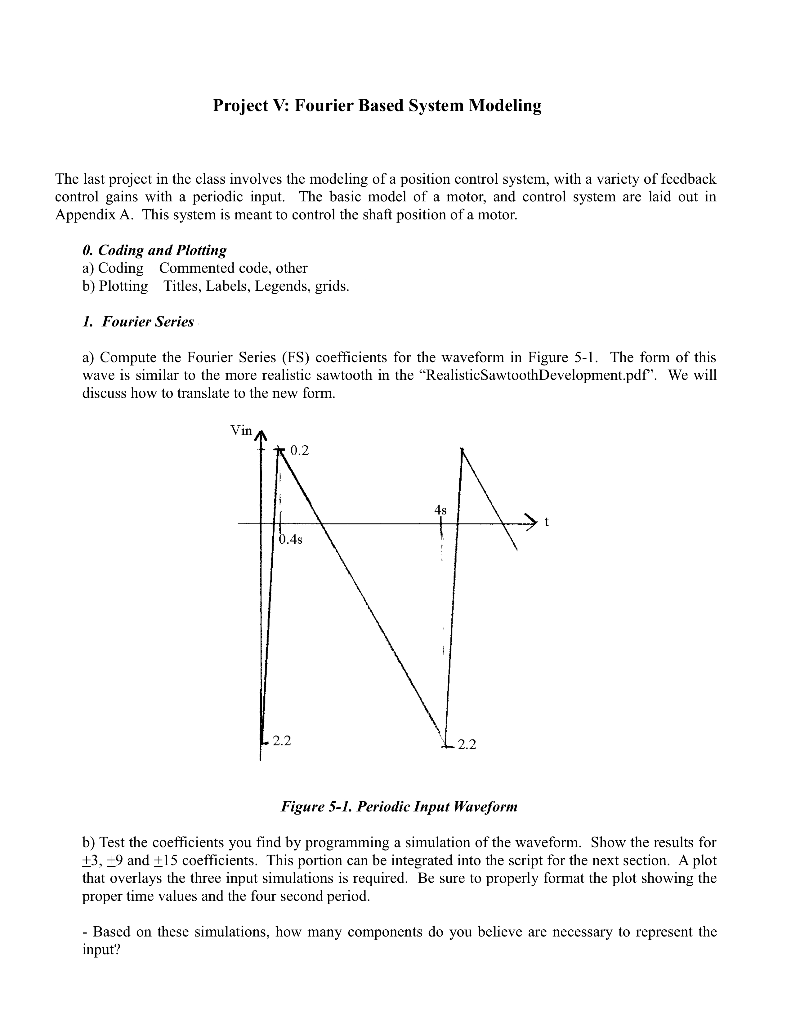Project V: Fourier Based System Modeling The last project in the class involves the modeling of a position control system, with a variety of fcedback control gains with a periodic input. The basic model of a motor, and control system are laid out in Appendix A. This system is meant to control the shaft position of a motor. 0. Coding and Plotting a) Coding Commented code, other b) Plotting Titles, Labels, Legends, grids. I. Fourier Series a) Compute the Fourier Series (FS) coefficients for the waveform in Figure 5-1. The form of this wave is similar to the more realistic sawtooth in the "RealisticSawtoothDevelopment.pdf". We will will discuss how to translate to the new Vin 0.2 4s 0.4s 2.2 2.2 Figure 5-1. Periodic Input Waveform b) Test the coefficients you find by programming a simulation of the waveform. Show the results for 13,-9 and+15 coefficients. This portion can be integrated into the script for the next section. A plot that overlays the three input simulations is required. Be sure to properly format the plot showing the proper time values and the four second period Based on these simulations, how many components do you believe are necessary to represent the input?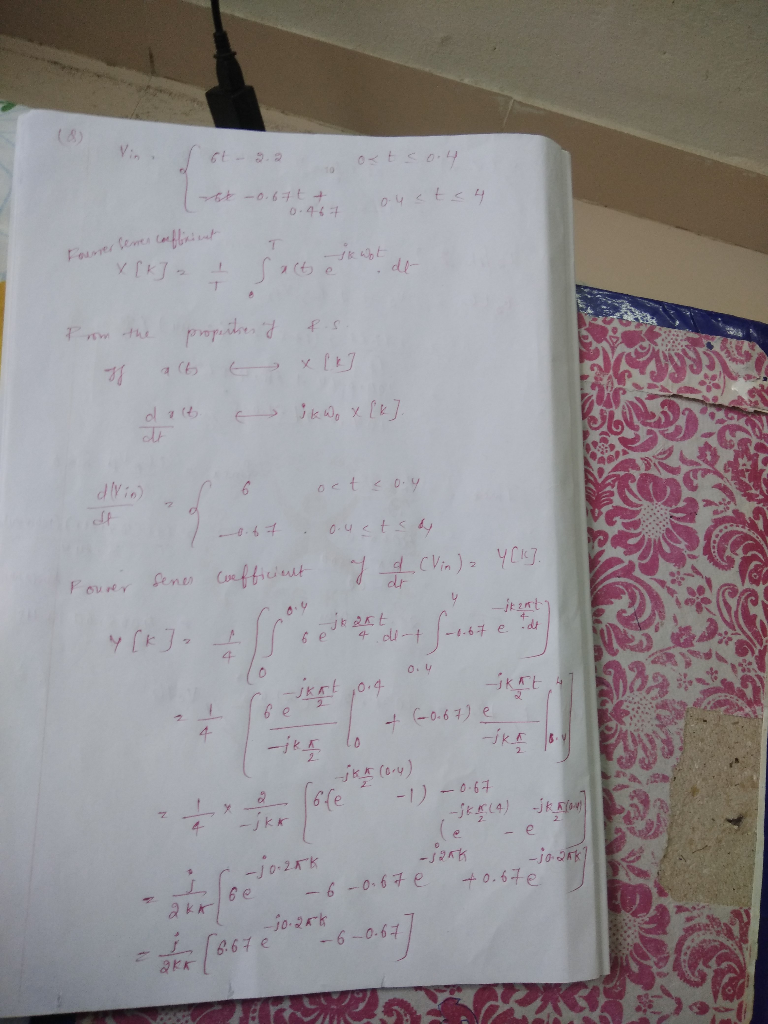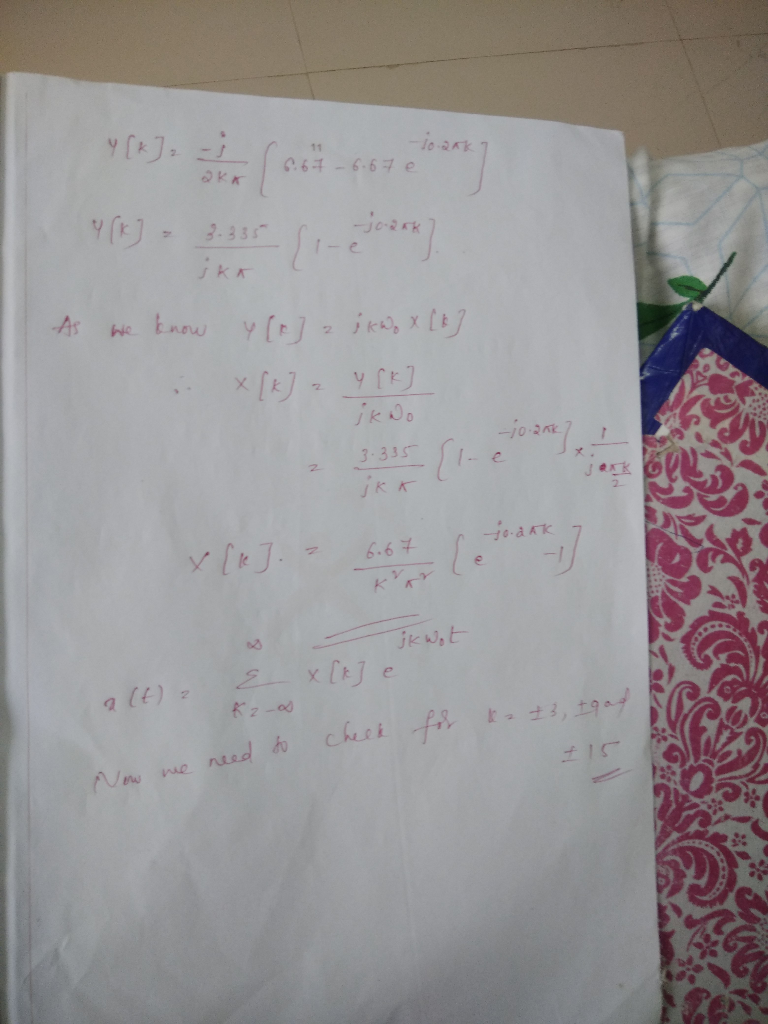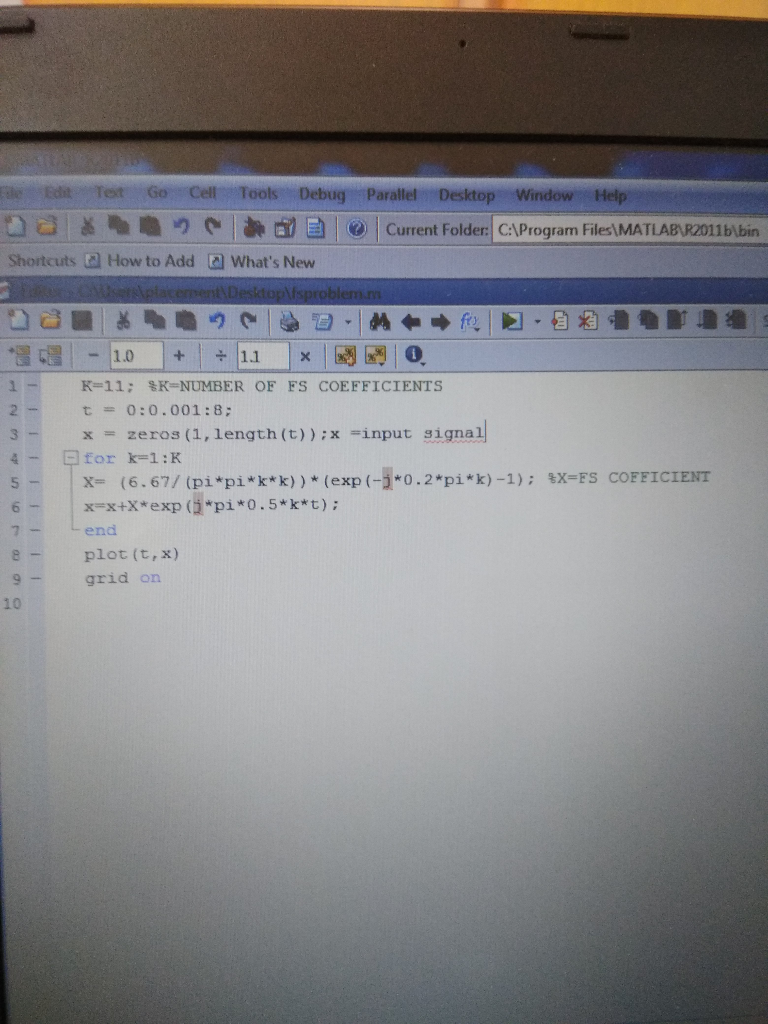Minimum of 15 coefficients are enough to get the input waveform..

kindly check with above code..

thank you..

##### Add Answer of: MATLAB ONLY MATLAB ONLY MATLAB ONLY MATLAB ONLY MATLAB ONLY MATLAB ONLY MATLAB ONLY MATLAB ONLY....... Project V: Fourier Based System Modeling The last project in the class involves the modeling of a...
Similar Homework Help Questions
• ### B Frequency Response Modeling Frequency response modeling of a linear system is based on the prem...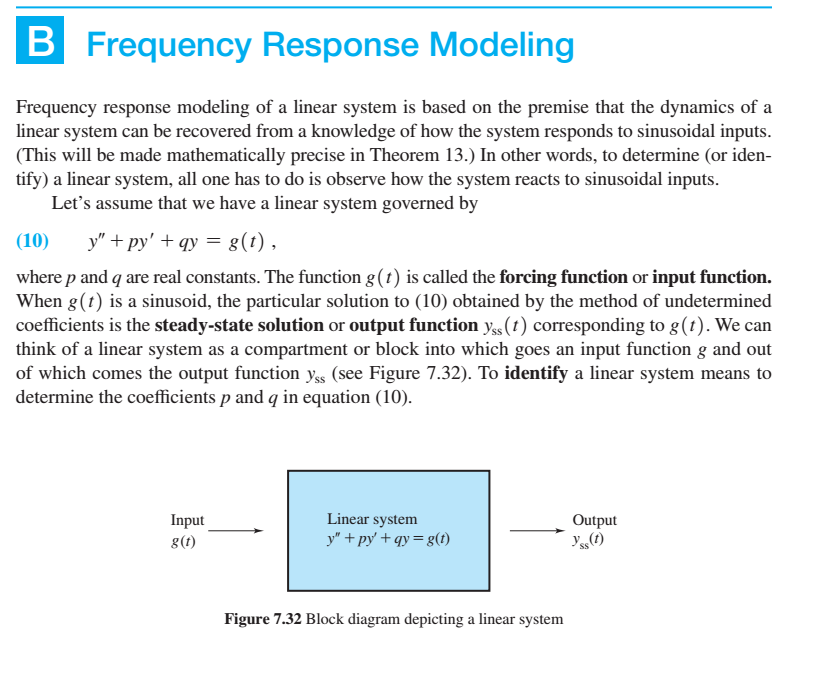Please explain every step as clearly and detailed as possible. B Frequency Response Modeling Frequency response modeling of a linear system is based on the premise that the dynamics of a linear system can be recovered from a knowledge of how the system responds to sinusoidal inputs. (This will be made mathematically precise in Theorem 13.) In other words, to determine (or iden- tify) a linear system, all one has to do is observe how the system reacts to sinusoidal...

• ### During lab 4, we have seen numerical implementation of Fourier Series for periodic signals. As first part of this assignment, you need to write a Matlab function that would take an array representing...

During lab 4, we have seen numerical implementation of Fourier Series for periodic signals. As first part of this assignment, you need to write a Matlab function that would take an array representing a single period of a signal (x), corresponding time array (t), and return the Fourier Series coefficients (Ck) in exponential form. The function should also be able to take two (2) optional input arguments: number of Fourier coefficients (Nk) and plot option (p). Use the template ‘fourier_series_exp.m’...

• ### Write as MATLAB code with comments thank you. The system in Figure 3 comprises a motor and a contoller. The performance requirements entail a steady state error for ramp input r(t) Ct, smaller than 0...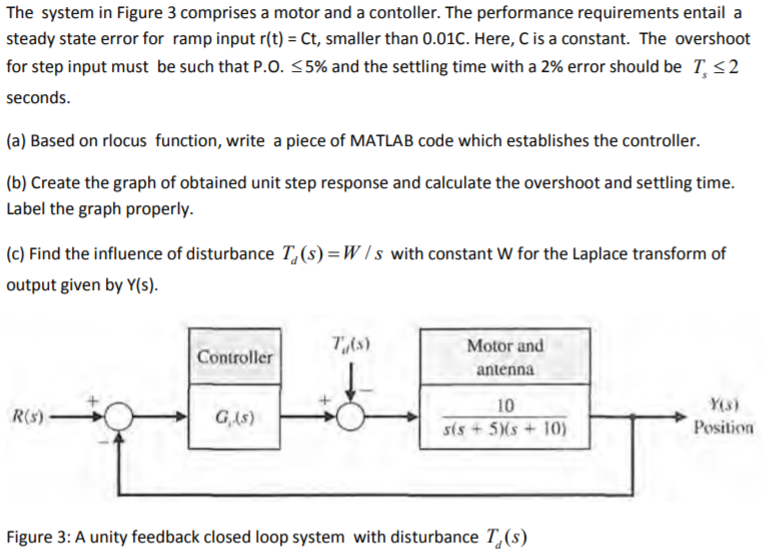Write as MATLAB code with comments thank you. The system in Figure 3 comprises a motor and a contoller. The performance requirements entail a steady state error for ramp input r(t) Ct, smaller than 0.01C. Here, C is a constant. The overshoot for step input must be such that P.0.S 5% and the settling time with a 2% error should be T. 2 seconds. (a) Based on rlocus function, write a piece of MATLAB code which establishes the controller. (b)...

• ### please solve as matlab code. The system in Figure 3 comprises a motor and a contoller. The performance requirements entail a steady state error for ramp input r(t) Ct, smaller than 0.01C. Here, C...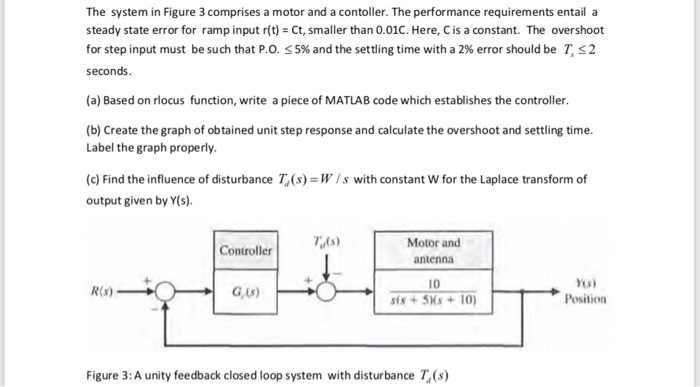please solve as matlab code. The system in Figure 3 comprises a motor and a contoller. The performance requirements entail a steady state error for ramp input r(t) Ct, smaller than 0.01C. Here, C is a constant. The overshoot for step input must be such that P.0. 5% and the settling time with a 2% error should be T, 2 seconds (a) Based on rlocus function, write a piece of MATLAB code which establishes the controller. (b) Create the graph...

• ### Using MATLAB, duplicate to the two graphs shown in the figure below Requirements 1. Show the MATLAB code used to plot both functions 5cos (nt-ng) (V) 5 e-"X cos (2nft-2nx/A) (V) a. y b- Use the f...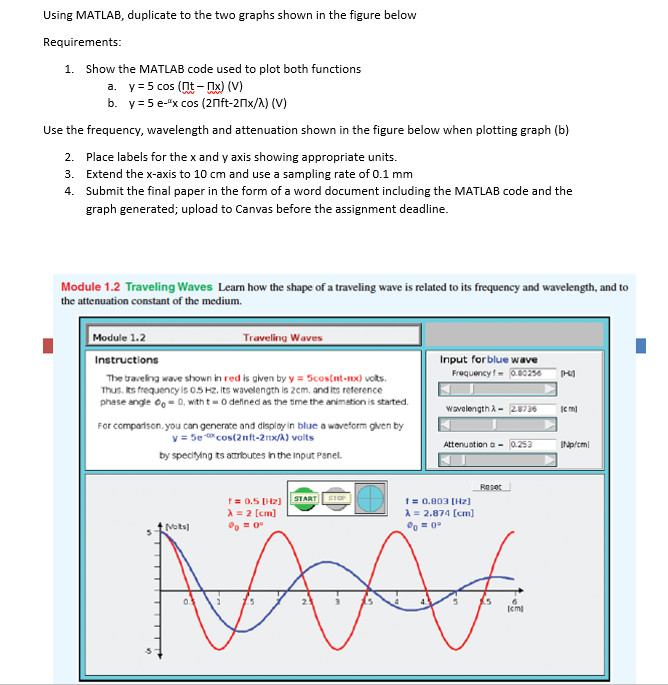Using MATLAB, duplicate to the two graphs shown in the figure below Requirements 1. Show the MATLAB code used to plot both functions 5cos (nt-ng) (V) 5 e-"X cos (2nft-2nx/A) (V) a. y b- Use the frequency, wavelength and attenuation shown in the figure below when plotting graph (b) 2. 3. 4. Place labels for the x and y axis showing appropriate units Extend the x-axis to 10 cm and use a sampling rate of 0.1 mm Submit the final...

• ### Please solve it with step by step MATLAB code, thank you! Suppose that a system is shown in Figure 2. Based on for loop, write a piece of MATLAB code to calculate the closed loop poles for 03K35 and...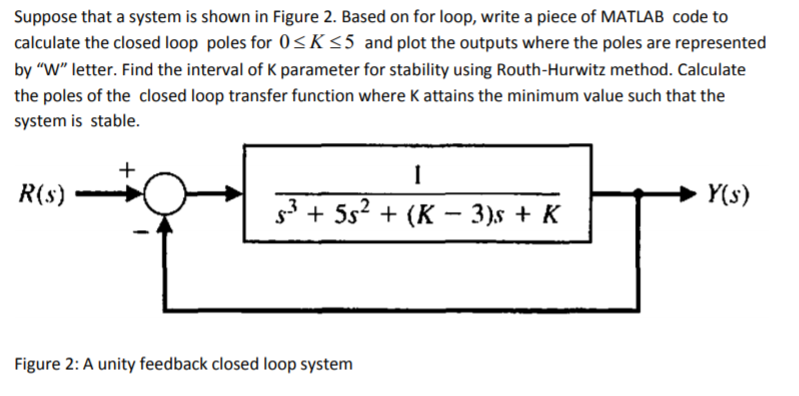Please solve it with step by step MATLAB code, thank you! Suppose that a system is shown in Figure 2. Based on for loop, write a piece of MATLAB code to calculate the closed loop poles for 03K35 and plot the outputs where the poles are represented by "W" letter. Find the interval of K parameter for stability using Routh-Hurwitz method. Calculate the poles of the closed loop transfer function where K attains the minimum value such that the system...

• ### Consider the electromechanical dynamic system shown in Figure 1(a). It consists of a cart of mass...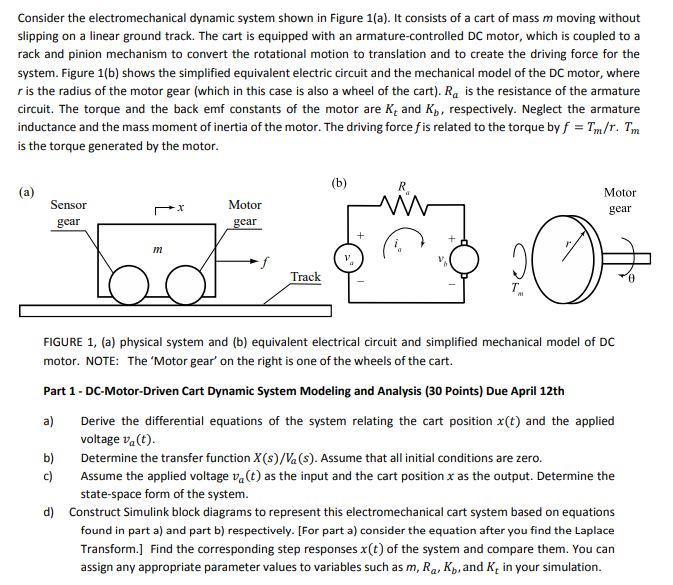This assignment is for my Engr dynamics systems class. Consider the electromechanical dynamic system shown in Figure 1(a). It consists of a cart of mass m moving without slipping on a linear ground track. The cart is equipped with an armature-controlled DC motor, which is coupled to a rack and pinion mechanism to convert the rotational motion to translation and to create the driving force for the system. Figure 1(b) shows the simplified equivalent electric circuit and the mechanical model...

• ### ONLY NEED SOLUTION TO QUESTION 4 PLEASE, THANK YOU (PLEASE MAKE SURE TO PLOT THE SOLUTION IN MATLAB) Part A (Based off week 7 Workshop content) Consider the RLC circuit where R = 5, C = 1, L = 4 and...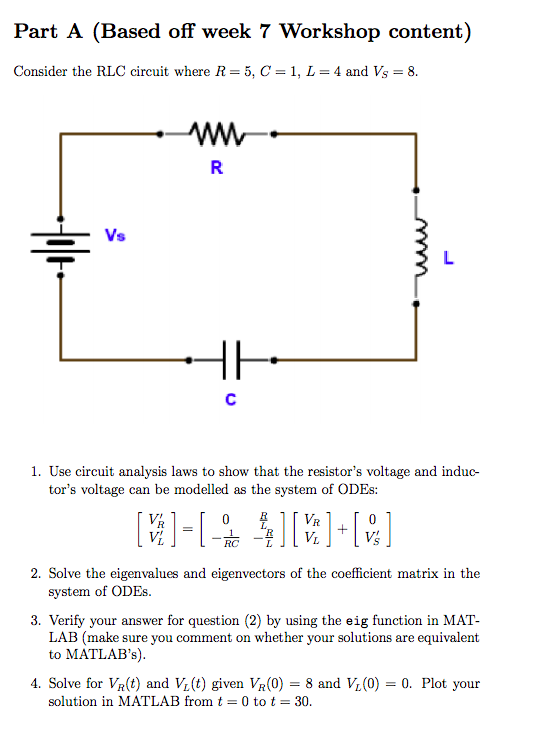ONLY NEED SOLUTION TO QUESTION 4 PLEASE, THANK YOU (PLEASE MAKE SURE TO PLOT THE SOLUTION IN MATLAB) Part A (Based off week 7 Workshop content) Consider the RLC circuit where R = 5, C = 1, L = 4 and Vs = 8. 1. Use circuit analysis laws to show that the resistor's voltage and induc tor's voltage can be modelled as the system of ODEs: L RC i the system of ODEs 3. Verify your answer for question...

• ### [MATLAB Coding] A four-bar linkage system is shown above. The first link, a, is an input link (crank) of length 1. The second link, b, is a coupler link of length 2. The third link, c, is an output l...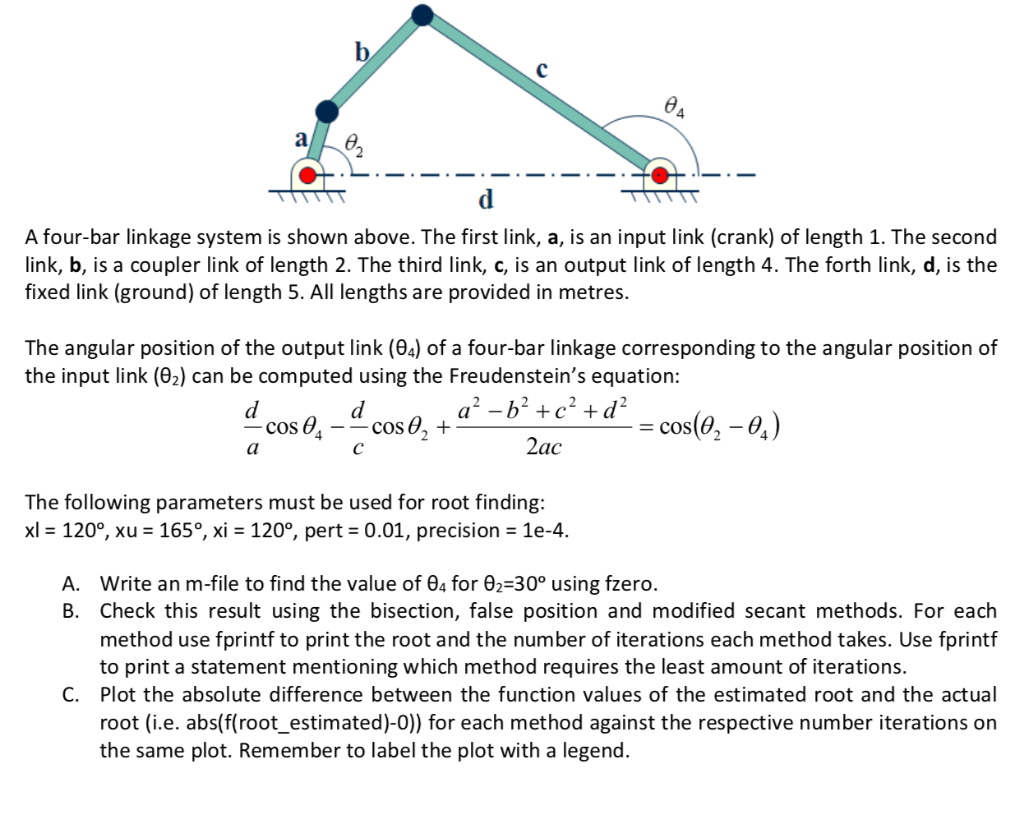[MATLAB Coding] A four-bar linkage system is shown above. The first link, a, is an input link (crank) of length 1. The second link, b, is a coupler link of length 2. The third link, c, is an output link of length 4. The forth link, d, is the fixed link (ground) of length 5. All lengths are provided in metres. Please answer the whole question in MATLAB coding. 4 A four-bar linkage system is shown above. The first link,...

• ### PROBLEM 2 Suppose that a system is shown in Figure 2. Based on for loop, write a piece of MATLAB code to calculate the closed loop poles for 0sKs5 and plot the outputs where the poles are represented...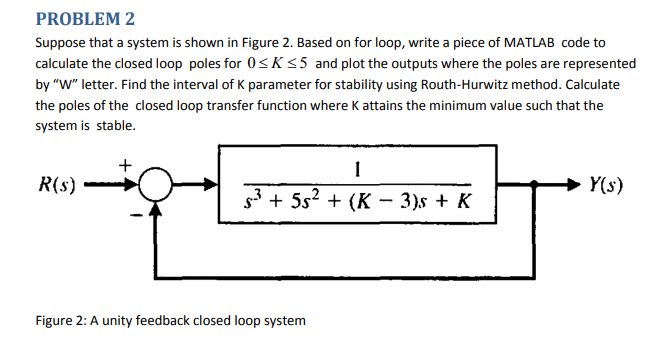PROBLEM 2 Suppose that a system is shown in Figure 2. Based on for loop, write a piece of MATLAB code to calculate the closed loop poles for 0sKs5 and plot the outputs where the poles are represented by "W" letter. Find the interval of K parameter for stability using Routh-Hurwitz method. Calculate the poles of the closed loop transfer function where K attains the minimum value such that the system is stable. R(s) 52(K - 3)s + K Figure...